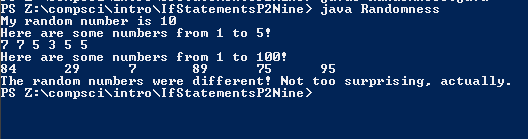# Assignment #53 Randomness

## Code

```        /// Name: Lacey Reese
/// Period: 5
/// Program Name:  Randomness
/// File Name:  Randomness.java
/// Date Finished 11/20/2015

import java.util.Random;

public class Randomness
{

public static void main( String[] args )
{

Random r = new Random();

int x = 1 + r.nextInt(10);

//When I deleted 1+ the range was 0 through 4
// When I added 3+ that range is from 3 to 7
// Putting 12353 or the seed number caused it to produce the same number.
// When I put any random seed number, it produced the same number

System.out.println( "My random number is " + x );

System.out.println( "Here are some numbers from 1 to 5!" );
System.out.print( 3 + r.nextInt(5) + " " );
System.out.print( 3 + r.nextInt(5) + " " );
System.out.print( 3 + r.nextInt(5) + " " );
System.out.print( 3 + r.nextInt(5) + " " );
System.out.print( 3 + r.nextInt(5) + " " );
System.out.print( 3 + r.nextInt(5) + " " );
System.out.println();

System.out.println( "Here are some numbers from 1 to 100!" );
System.out.print( 1 + r.nextInt(100) + "\t" );
System.out.print( 1 + r.nextInt(100) + "\t" );
System.out.print( 1 + r.nextInt(100) + "\t" );
System.out.print( 1 + r.nextInt(100) + "\t" );
System.out.print( 1 + r.nextInt(100) + "\t" );
System.out.print( 1 + r.nextInt(100) + "\t" );
System.out.println();

int num1 = 1 + r.nextInt(10);
int num2 = 1 + r.nextInt(10);

if ( num1 == num2 )
{
System.out.println( "The random numbers were the same! Weird." );
}

if ( num1 != num2 )
{
System.out.println( "The random numbers were different! Not too surprising, actually." );
}

}
}

Picture of the output
//```